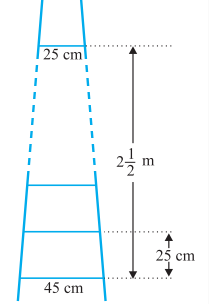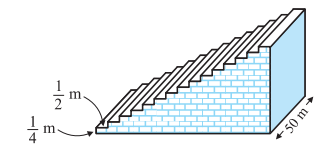# NCERT Solutions for Class 10 Maths Chapter 5 Exercise 5.4

In this page we have NCERT Solutions for Class 10th Maths: Chapter 5 - Arithmetic Progressions for EXERCISE 5.4 . Hope you like them and do not forget to like , social_share and comment at the end of the page.
• The sum of the first n terms of an AP is given by :
$S =\frac {n}{2} [2a +(n-1)d]$
• If l is the last term of the finite AP, say the nth term, then the sum of all terms of the AP is given by :
$S =\frac {n}{2} [a +l]$
• If a ,b,c are in A.P.then
$b= \frac {a+c}{2}$
And b is called the Arithmetic Mean

## NCERT Solutions for Class 10 Maths Chapter 5 - Arithmetic Progressions Exercise 5.4

Question 1.
Which term of the AP : 121, 117, 113, . . ., is its first negative term? [Hint : Find n for $a_n > 0$]
Solution
Given, The AP series is 121, 117, 113, . . .,
first term, a = 121
Common difference, d = 117-121= -4
nth term of the AP is given by
$a_n= a + (n-1)d$ So nth term of the given AP will be,
$a_n=121+ (n- 1)(-4)$
$=125-4n$
To find the first negative term of the series,$a_n > 0$
So,
$125-4n > 0$ $n > 125/4$
$n > 31.25$
Hence,the first negative term of the series is 32th term.

Question 2.
The sum of the third and the seventh terms of an AP is 6 and their product is 8. Find the sum of first sixteen terms of the AP.
Solution:
Let a and d be the First term and common difference Also nth term of the AP is given by
$a_n= a + (n-1)d$ From the given statements, we can write,
$a_3 + a_7= 6$
$a+ 2d + a + 6d =6$
$2a + 8d =6$
$a + 4d =3$
$a = 3 -4d$ --(1)
And
$a_3 \times a_7= 8$
$(a+ 2d)(a+ 6d) =9$ Now Substituting the values of a from (1) $(3 - 4d + 2d) \times (3 - 4d + 6d) = 8$
$(3 - 2d) \times (3 + 2d) = 8$
$3^2- (2d)^2= 8$
$4d^2= 1$
d = 1/2 or -1/2
Now, by putting both the values of d, we get,
When d= 1/2 $a = 3 - 4d = 3 - 4(\frac {1}{2}) = 3 - 2 = 1$
Now We know, the sum of nth term of AP is;
$S_n=\frac {n}{2}[2a+ (n- 1)d]$
$S_{16}=\frac {16}{2}[2+ (16- 1)\frac {1}{2}] = 8(2 + \frac {15}{2}) = 76$
When d= 1/2 $a = 3 - 4d = 3 - 4(-\frac {1}{2}) = 3+2 = 5$
Then, the sum of first 16 terms are;
$S_{16}=16/2[10+ (16- 1)(-\frac {1}{2})] = 8(\frac {5}{2})=20$

Question 3.
A ladder has rungs 25 cm apart. The rungs decrease uniformly in length from 45 cm at the bottom to 25 cm at the top. If the top and the bottom rungs are $2 \frac {1}{2}$m apart, what is the length of the wood required for the rungs? [Hint : Number of rungs = 250/25 + 1 ].Solution
Given,
Distance between the rungs of the ladder is 25cm.
Distance between the top rung and bottom rung of the ladder is=$2 \frac {1}{2}$ m = 250cm
Therefore, total number of rungs = $\frac {250}{25} + 1 = 11$
As per the question, the ladder has rungs in decreasing order uniformly from top to bottom.So, Lenght of the each rungs forms an A.P
And the length of the wood required for the rungs will be equal to the sum of the terms of AP series formed.
So,
First term, a = 45
Last term,l =25
Number of terms, n = 11
Now, sum of nth terms is equal to,
$S_n= \frac {n}{2}(a+l)$
$S_n= \frac {11}{2}(45+25) = 385 cm$
Hence, the length of the wood required for the rungs is 385cm.

Question 4.
The houses of a row are numbered consecutively from 1 to 49. Show that there is a value of x such that the sum of the numbers of the houses preceding the house numbered x is equal to the sum of the numbers of the houses following it. Find this value of x. [Hint : $S_{x - 1} = S_{49} - S_x$ ]
Solution:Given,
Row houses are numbers from 1,2,3,4,5…….49.
So it is an A.P.
First term, a = 1
Common difference, d=1
Now Sum of nth term of AP
$S_n= \frac {n}{2}[2a+(n-1)d]$
Now as per Question
$S_{x - 1} = S_{49} - S_x$
$\frac {(x-1)}{2} [2+ (x-1-1)\times 1] = \frac {49}{2} [2 + (49-1) \times 1] - \frac {x}{2} [2+ (x-1)\times 1]$
$\frac {(x-1)}{2} [2 + x-2] = \frac {49}{2} [ 50] - \frac {x}{2} [2+ x-1]$
$\frac {x(x-1)}{2} = 1225 - \frac {x}{2} [x+1]$
$x^2 -x = 2450 - x^2 -x$
$2x^2 = 2450$
$x^2 = 1225$
$x= 35$
Hence, the value of x is 35.

Question 5.
A small terrace at a football ground comprises of 15 steps each of which is 50 m long and built of solid concrete. Each step has a rise of 1/4 m and a tread of 1/2 m. Calculate the total volume of concrete required to build the terrace. [Hint : Volume of concrete required to build the first step = $\frac {1}{4} \times \frac {1}{2} \times 50$].Solution:Given,
Volume of concrete required to build the first step = $\frac {1}{4} \times \frac {1}{2} \times 50 = \frac {1}{4} \times 25 m^3$
Volume of concrete required to build the second step = $\frac {2}{4} \times \frac {1}{2} \times 50 = \frac {2}{4} \times 25 m^3$
Volume of concrete required to build the third step = $\frac {3}{4} \times \frac {1}{2} \times 50 = \frac {3}{4} \times 25 m^3$
---
Volume of concrete required to build the second step = $\frac {15}{4} \times \frac {1}{2} \times 50 = \frac {15}{4} \times 25 m^3$
We can see that these number form the A.P
First Term (a) = $\frac {1}{4} \times 25$
Common Difference (d) =$\frac {2}{4} \times 25 - \frac {1}{4} \times 25= \frac {1}{4} \times 25$
Last Term (l) = $\frac {15}{4} \times 25$
Hence total Volume of Concrete requird will the sum of the A.P
$S_{15} = \frac {15}{2} [2 \times \frac {15}{4} \times 25 + (15 -1) \times \frac {15}{4} \times 25]$
$=750 m^3$

## Summary

1. NCERT Solutions for Class 10th Maths: Chapter 5 - Arithmetic Progressions Ex 5.4 has been prepared by Expert with utmost care. If you find any mistake.Please do provide feedback on mail.You can download this as pdf
2. This chapter 5 has total 4 Exercise 5.1 ,5.2,5.3 and 5.4. This is the fourth exercise in the chapter.You can explore previous exercise of this chapter by clicking the link below

Go back to Class 10 Main Page using below links

### Practice Question

Question 1 What is $1 - \sqrt {3}$ ?
A) Non terminating repeating
B) Non terminating non repeating
C) Terminating
D) None of the above
Question 2 The volume of the largest right circular cone that can be cut out from a cube of edge 4.2 cm is?
A) 19.4 cm3
B) 12 cm3
C) 78.6 cm3
D) 58.2 cm3
Question 3 The sum of the first three terms of an AP is 33. If the product of the first and the third term exceeds the second term by 29, the AP is ?
A) 2 ,21,11
B) 1,10,19
C) -1 ,8,17
D) 2 ,11,20